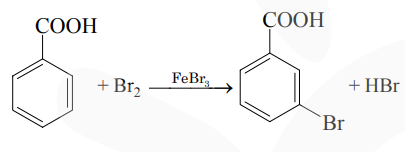# Prove the following

Question:Consider the above reaction where $6.1 \mathrm{~g}$ of benzoic acid is used to get $7.8 \mathrm{~g}$ of m-bromo benzoic acid. The percentage yield of the product is_________.

(Round off to the Nearest integer)

[Given : Atomic masses : $\mathrm{C}=12.0 \mathrm{u}, \mathrm{H}: 1.0 \mathrm{u}$, $\mathrm{O}: 16.0 \mathrm{u}, \mathrm{Br}=80.0 \mathrm{u}]$

Solution:

Moles of Benzoic acid $=\frac{6.1}{122}$

$=$ moles of m-bromobenzoic acid

So, weight of m-bromobenzoic acid

$=\frac{6.1}{122} \times 201 \mathrm{gm}$

$=10.05 \mathrm{gm}$

$\%$ yield $=\frac{\text { Actual weight }}{\text { Theoretical weight }} \times 100$

$=\frac{7.8}{10.05} \times 100$

$=77.61 \%$